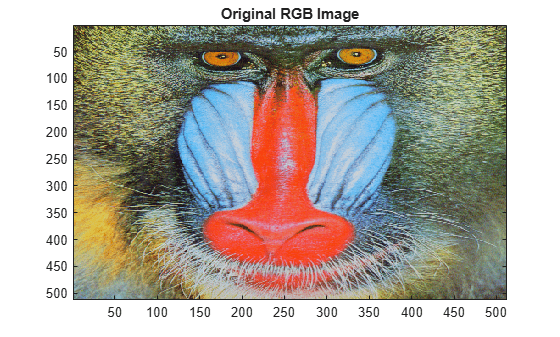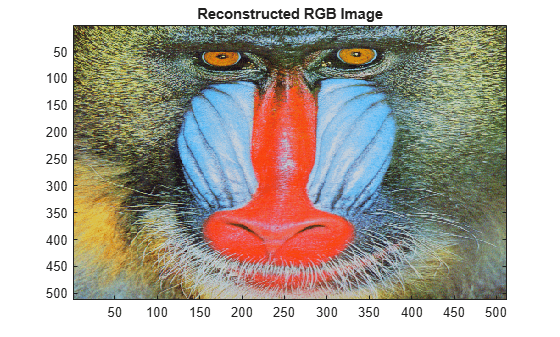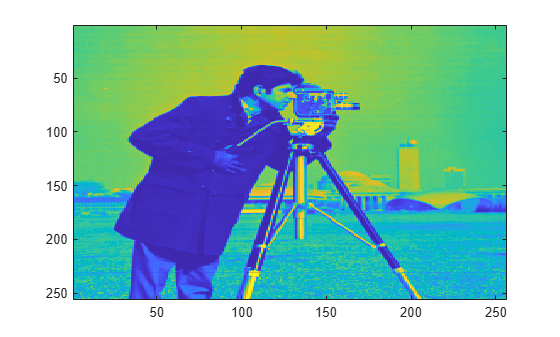ihaart2

Inverse 2-D Haar wavelet transform

Description

example

xrec = ihaart2(a,h,v,d) returns the inverse 2-D Haar transform, xrec, for the approximation coefficients, a, and the horizontal, vertical, and diagonal detail coefficients, h, v, and d. All the inputs, a, h, v, and d, are outputs of haart2.

example

xrec = ihaart2(a,h,v,d,level) returns the inverse 2-D Haar transform at the specified level.

example

xrec = ihaart2(___,integerflag) specifies how the inverse 2-D Haar transform handles integer-valued data, using any of the previous syntaxes.

Examples

collapse all

Obtain the inverse 2-D Haar transform of image and view the reconstructed image.

Load the image and obtain its 2-D Haar transform.

[a,h,v,d] = haart2(im);

Use the inverse 2-D Haar transform to reconstruct the image.

xrec = ihaart2(a,h,v,d);

Compare the original and reconstructed images.

imagesc(im)
title('Original RGB Image')figure
imagesc(uint8(xrec))
title('Reconstructed RGB Image')Obtain the 2-D Haar transform of an image limiting the transform to 2 levels.

Load and view the image of a cameraman.

imagesc(im)Obtain the 2-D Haar transform using the default maximum number of levels.

[a,h,v,d] = haart2(im);

Reconstruct the image using the inverse 2-D Haar transform and view the image. Notice the near-perfect reconstruction.

xrec = ihaart2(a,h,v,d);
imagesc(xrec)Reconstruct and view the image using the inverse 2-D Haar transform, limited to level 2. Level 2 corresponds to the fourth scale because scale is defined as ${2}^{j}$, where j is the level.

xrec1 = ihaart2(a,h,v,d,2);
imagesc(xrec1)Using fewer levels returns the average of the original image at level 2.

Obtain the 2-D Haar transform of an image limiting the transform to integer data.

Load the image of a cameraman.

Obtain the 2-D Haar transform using the 'integer' flag.

[a,h,v,d]=haart2(im,'integer');

Reconstruct the image using the inverse 2-D Haar transform and view the image.

xrec = ihaart2(a,h,v,d,'integer');
imagesc(xrec)Use integer data when you need to reduce the amount of memory used compared to noninteger data.

Input Arguments

collapse all

Approximation coefficients, specified as a scalar or matrix of coefficients, depending on the level to which the 2-D Haar transform was calculated. a is an output from the haart2 function. Approximation, or scaling, coefficients are a lowpass representation of the input. If a and the elements of h, v, and d, are vectors, xrec is a vector. If a and the elements of h, v, and d are matrices, xrec is a matrix, where each column is the inverse 2-D Haar transform of the corresponding columns in a and h, v, or d.

Data Types: single | double

Horizontal detail coefficients by level, specified as a matrix or cell array of matrices. h is an output from the haart2 function. If h is a matrix, the 2-D Haar transform was computed only down to one level coarser in resolution.

Data Types: single | double

Vertical detail coefficients by level, specified as a matrix or cell array of matrices. v is an output from the haart2 function. If v is a matrix, the 2-D Haar transform was computed only down to one level coarser in resolution.

Data Types: single | double

Diagonal detail coefficients by level, specified as a matrix or cell array of matrices. d is an output from the haart2 function. If d is a matrix, the 2-D Haar transform was computed only down to one level coarser in resolution.

Data Types: single | double

Maximum level to which to invert the Haar transform, specified as a nonnegative integer. If h is a cell array, level is less than or equal to length(h)-1. If h is a vector or matrix, level must equal 0 or be unspecified.

Integer-valued data handling, specified as either 'noninteger' or 'integer'. 'noninteger' does not preserve integer-valued data in the 2-D Haar transform, and 'integer' preserves it. The 'integer' option applies only if all elements of inputs, a, h, v, and d, are integer-valued. The inverse 2-D Haar transform algorithm, however, uses floating-point arithmetic.

Output Arguments

collapse all

2-D Haar wavelet transform, returned as a matrix.

Data Types: single | double

Extended Capabilities

GPU ArraysAccelerate code by running on a graphics processing unit (GPU) using Parallel Computing Toolbox™.